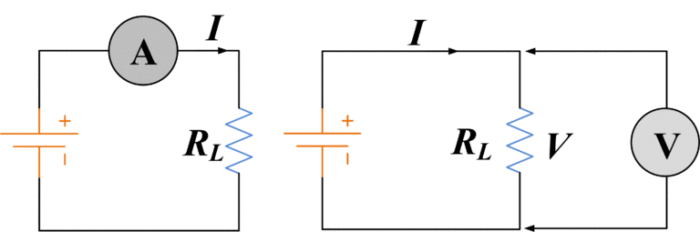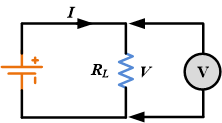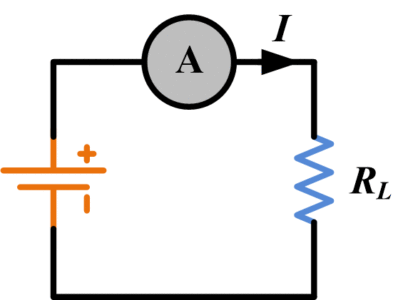# Ammeter vs Voltmeter | Difference between Ammeter and Voltmeter

Want create site? Find Free WordPress Themes and plugins.

Devices like ammeter and voltmeter are employed in order to measure electricity. Both of these devices are based on the galvanometer which is employed to detect small current. An ammeter is a device which is used to measure the “current” (in ampere, micro-ampere, and milli-ampere) through a conductor whereas a voltmeter is a device which is used to measure the “voltage” (in volts) between two points or terminals. Both the devices act very differently in terms of operation as well as circuit placement.The key differences between Ammeter and Voltmeter are discussed in this tutorial on the basis of certain important factors such as connection with appliances, uses, safety, resistance, ideal behavior, and measuring units.The following table points out the key Differences between Ammeter and Voltmeter.

## Difference between Ammeter and Voltmeter

CharacteristicsAmmeterVoltmeter
UseMeasures current through an applianceMeasures potential difference between two points
Measuring unitMeasures current in amperes or even smaller units (mA, μ A)Potential difference is measured in volts.
SafetyLow resistance to electricity so must not be connected straight across the supplyHigh resistance to electricity so be able to be connected straight across the supply
Amount of currentLarge amount of current passes throughVery small amount of current passes through
Connection with applianceResistanceLow resistance instrumentHigh resistance instrument
Ideal behaviorResistance of an ideal ammeter is zeroResistance of an ideal voltmeter is infinity
CircuitCircuit must be disconnected from the power source in order to attach the ammeterCircuit does not need to be disconnected to attach the voltmeter
Representation## How to connect ammeter in a circuit

An ammeter must be connected so that the current to be measured flows through the instrument. Consequently, Ammeters are always connected in series with the component in which current is to be measured, as shown in the figure.An ammeter must have a very low resistance so that it does not affect the current level when connected to a circuit.

How to connect voltmeter in a circuit

A voltmeter must be connected so that the voltage to be measured appears at the voltmeter terminals. Consequently, A voltmeter is always connected in parallel with the component that is to have its voltage measured, as shown in the figure.A voltmeter must have a very high resistance so that it does not affect the voltage level when connected to a circuit.

Did you find apk for android? You can find new Free Android Games and apps.